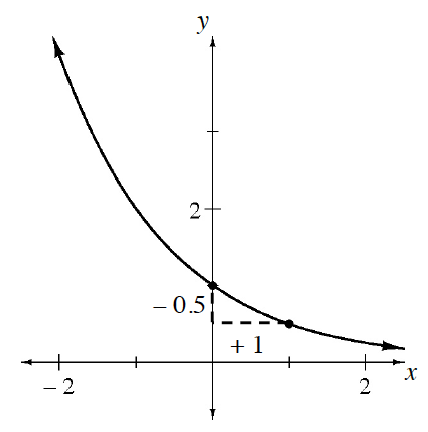### Home > INT1 > Chapter 8 > Lesson 8.2.2 > Problem8-111

8-111.

Use the graph below to write a possible equation in $y = ab^x$ form.What is the value of $a$, when $x = 0$?

What is the multiplier ($b$)? Substitute $(x, y)$ from a different point, and solve for $b$.